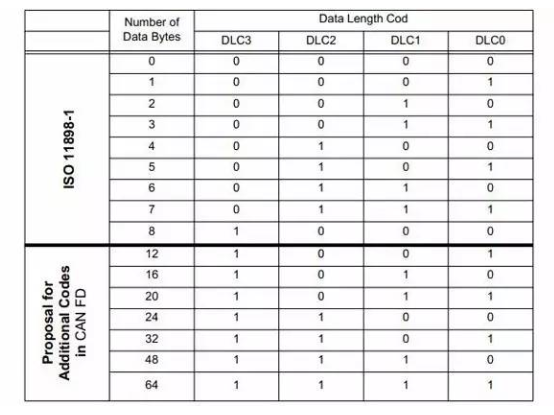# Difference between CAN and CAN-FD

According to the standard frame structure defined by can specification iso11898-2, a frame message can only transmit 64 bits (8 bytes) of data at most. In the best case, the bus load can reach about 70%, but if the message data is less than 8 bytes, the bus load may be smaller. We may ask why we can't raise the baud rate of can standard? In fact, there is a very important factor. Can uses arbitration mechanism to determine the priority of can message transmission of different nodes. During the arbitration period, all nodes on the same bus must arrive in a bit time. On the other hand, electrical signals have a limited propagation speed. From a lot of experience, the longest distance of CAN bus running at 1mbit / S is 40m. The higher the baud rate, the shorter the communication distance.

Main differences between CAN FD and CAN:

There are two main differences between CAN-FD and CAN

1. Variable rate

CAN-FD adopts two bit rates: the variable rate is from the BRS bit in the control field to the front of the ACK field (including CRC separator), and the rest is the rate used by the original can bus. Each of the two rates has a set of bit time definition registers. In addition to using different bit time units TQ, the bit time allocation ratio of each segment can also be different.

2. New data field length

CAN-FD greatly expands the length of the data field. The DLC supports 64 bytes at most. When the DLC is less than or equal to 8, it is the same as the original can bus. When the DLC is greater than 8, there is a nonlinear growth, so the maximum data field length can reach 64 bytes.

Introduction of CAN FD

1. CAN-FD data frame format

EDL bit, BRS bit and ESI bit are added to the control field of CAN-FD data frame. A new DLC encoding method and a new CRC algorithm are adopted (CRC field is extended to 21 bits). CAN-FD data frame format is shown in Figure 3.

2. Introduction of new added bit

EDL bit: (extended data length) the reserved bit r in the original CAN data frame. The function of this bit is:

Recessive: indicates CAN-FD message (using new DLC coding and CRC algorithm)

Explicit: indicates can message

BRS bit: (bit rate switch) the function of this bit is:

Recessive: it means conversion variable rate

Dominant: indicates no conversion rate

ESI (error state indicator), the function of this bit is:

Recessive: indicates that the sending node is in an error passive state

Explicit: indicates that the sending node is in the active error state

EDL bit can indicate can message or can-fd message; BRS represents bit rate conversion. When the bit is recessive, the conversion rate is used to transmit from BRS bit to CRC identifier, and other bit fields use standard bit rate. When the bit is dominant, it is transmitted at normal can-fd bus rate. By adding ESI bit, the status of current sending node can be easily known.

3. New CRC algorithm

Because of the interference of bit filling rule to CRC, the error frame miss detection rate of CAN bus is not up to the design intention. CAN-FD has changed the CRC algorithm, that is, CRC is calculated by bit stream with filling bits. In the check sum part, in order to avoid more than six consecutive bits, it is determined to add a padding bit to the first bit and every four bits after it to be segmented. The value of this padding bit is the inverse code of the previous bit. As a format check, if the padding bit is not the inverse code of the previous bit, an error will be dealt with. The CRC field of can-fd is extended to 21 bits. Because the length of data field has a large range of variation, different CRC should be applied according to the size of DLC to generate polynomial CRC_ 17, suitable for frames with frame length less than 210 bits, CRC_ 21, suitable for frames with frame length less than 1023 bits.

4. New DLC coding

A new DLC coding method is adopted in CAN-FD data frame. Linear rule is adopted when the data field length is 0-8 bytes, and nonlinear coding is used when the data field length is 12-64 bytes. As shown in Figure 5.